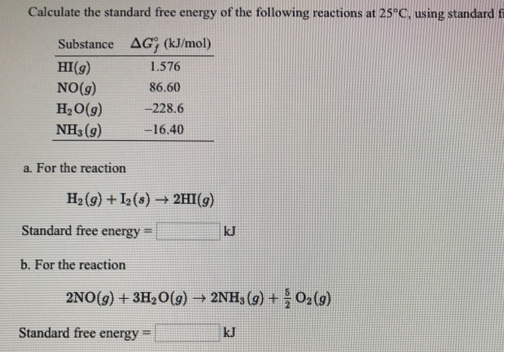# Problem: Calculate the standard free energy of the following reaction at 25 °C. a. For the reaction H2 (g) + I2 (s) → 2HI (g) b. For the reaction 2NO (g) + 3H2O (g) → 2NH3 (g) + 5/2O2 (g)

###### FREE Expert Solution
83% (344 ratings)###### Problem Details

Calculate the standard free energy of the following reaction at 25 °C.

a. For the reaction

H2 (g) + I(s) → 2HI (g)

b. For the reaction

2NO (g) + 3H2O (g) → 2NH(g) + 5/2O(g)